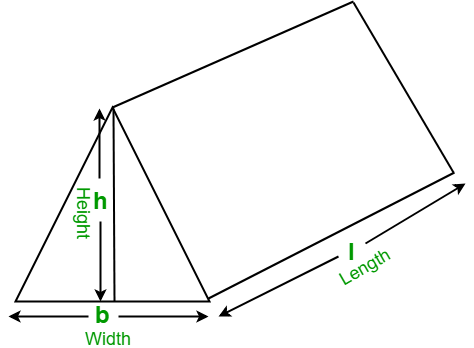Related Articles

# Program to find the Volume of a Triangular Prism

• Difficulty Level : Basic
• Last Updated : 17 Mar, 2021

Given the length, width, and height of a triangular prism, the task is to find the volume of the triangular prism.
Examples:

```Input:  l = 18, b = 12, h = 9
Output: Volume of triangular prism: 972

Input: l = 10, b = 8, h = 6
Output: Volume of triangular prism: 240```

In mathematics, a triangular prism is a three-dimensional solid shape with two identical ends connected by equal parallel lines. The triangular prism contains 5 faces, 9 edges, and 6 vertices.Formula to find the volume of triangular prism:

`Volume = ( l * b * h ) / 2 `

## C++

 `// CPP program to find the volume``// of the triangular prism``#include ``using` `namespace` `std;` `// function to find the Volume``// of triangular prism``float` `findVolume(``float` `l, ``float` `b, ``float` `h)``{``    ``// formula to find Volume``    ``float` `volume = (l * b * h) / 2;` `    ``return` `volume;``}` `// Driver Code``int` `main()``{``    ``float` `l = 18, b = 12, h = 9;` `    ``// function calling``    ``cout << ``"Volume of triangular prism: "``         ``<< findVolume(l, b, h);` `    ``return` `0;``}`

## Java

 `// Java program to find the volume``// of the triangular prism``import` `java.io.*;` `class` `GFG {` `    ``// function to find the Volume``    ``// of triangular prism``    ``static` `float` `findVolume(``float` `l, ``float` `b, ``float` `h)``    ``{``        ``// formula to find Volume``        ``float` `volume = (l * b * h) / ``2``;` `        ``return` `volume;``    ``}` `    ``// Driver code``    ``public` `static` `void` `main(String[] args)``    ``{``        ``float` `l = ``18``, b = ``12``, h = ``9``;` `        ``// function calling``        ``System.out.println(``"Volume of triangular prism: "``                           ``+ findVolume(l, b, h));``    ``}``}`

## Python3

 `# Python3 program to find the volume``# of the triangular prism` `# function to find the Volume``# of triangular prism``def` `findVolume(l, b, h) :` `    ``# formula to find Volume``    ``return` `((l ``*` `b ``*` `h) ``/` `2``)` `# Driver Code``l ``=` `18``b ``=` `12``h ``=` `9``    ` `# function calling``print``(``"Volume of triangular prism: "``,``                ``findVolume(l, b, h))`

## C#

 `// C# program to find the volume``// of the triangular prism``using` `System;` `class` `GFG {` `    ``// function to find the Volume``    ``// of triangular prism``    ``static` `float` `findVolume(``float` `l, ``float` `b, ``float` `h)``    ``{``        ``// formula to find Volume``        ``float` `volume = (l * b * h) / 2;` `        ``return` `volume;``    ``}` `    ``// Driver code``    ``static` `public` `void` `Main()``    ``{``        ``float` `l = 18, b = 12, h = 9;` `        ``// function calling``        ``Console.WriteLine(``"Volume of triangular prism: "``                          ``+ findVolume(l, b, h));``    ``}``}`

## PHP

 ``

## Javascript

 ``
Output:
`Volume of triangular prism: 972`

Attention reader! Don’t stop learning now. Get hold of all the important mathematical concepts for competitive programming with the Essential Maths for CP Course at a student-friendly price. To complete your preparation from learning a language to DS Algo and many more,  please refer Complete Interview Preparation Course.

My Personal Notes arrow_drop_up